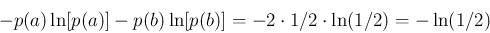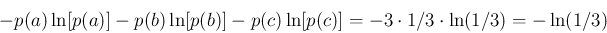# Considerations regarding the selection of the parameter r for the calculation of sample entropy

In the sample entropy algorithm, the parameter r is used to determine whether two data points, xi and xj, are distinguishable or not. If |xi - xj| r, then xi and xj are indistinguishable. Otherwise, they are “seen” as two different data points. In the MSEμ algorithm, the r value is traditionally chosen as a percentage of the SD of the original time series, typically a value between 15 and 20%. An advantage of choosing r in this way is the fact that two time series with different amplitudes but equal correlation properties are guaranteed to have the same sample entropy and the same MSE values. In fact, calculating sample entropy with an r value that is a percentage of the time series’ SD (e.g., 20%) is equivalent to calculating sample entropy with a fixed r value (0.2) of a previously normalized time series.

An important observation regarding the choice of r as a percentage of a time series’ SD is the fact that two RR interval data points, e.g., 625 and 633 ms, may be indistinguishable when analyzing a given time series and distinguishable when analyzing another one. Consider the time series A and B with SDs of 38 ms and 42 ms, respectively. If r = 20% of the time series’ SD, then r = 7.6 ms and r = 8.4 ms, for A and B, respectively. Therefore, in one case, the RR intervals 625 and 633 ms are “seen” as different (since |625 - 633| = 8.0 > 7.6), and in the other case, as indistinguishable, i.e., below the accepted level of noise (since |625 - 633| < 8.4). If one is interested in quantifying entropy of two time series at the same level of “resolution,” then one should choose a fixed (i.e., not dependent on SD) r value. However, in doing so, the following consideration should be kept in mind.

Let us consider two time series A and B taking values from the sets {a, b} and {a, b, c}, respectively. Assume that both time series are uncorrelated noise. For time series A, the probabilities of a and b are both 1/2; and for time series B, the probabilities of a, b and c are 1/3. To simplify the presentation, here we use Shannon entropy. For time series A, the entropy is:For time series B, the entropy is:The entropy for time series A is smaller than for time series B simply because the size of the alphabet of time series A is smaller (two symbols, a and b) than that of time series B (three symbols, a, b and c).

In conclusion, time series with a larger alphabet are more entropic than those with a smaller alphabet and identical correlation properties. Thus, when analyzing RR intervals time series with a fixed r value, if one finds that a given time series is more entropic than another, one cannot be sure what the source of the difference is. Observed differences in entropy could be due to differences in the degree of randomness of the time series, differences in their range of values (larger/smaller alphabets) or a combination of the two.

Note that the two approaches discussed for choosing the r value are both justifiable since they provide complementary information.

Our first application of MSE using a fixed r value was in a project whose objective was to help forecast the need for lifesaving interventions based solely on 15-min ECG signals: Cancio LC, Batchinsky AI, Baker WL, et al. Combat casualties undergoing lifesaving interventions have decreased heart rate complexity at multiple time scales. J Crit Care. 2013;28(6):1093-8.

In most studies employing MSEμ, the r value is set to a percentage of the SD of the C-G time series for the smallest scale included in the analysis. Typically, the smallest scale is scale one. Thus, the r value is a percentage of the original time series’ SD. This r value is then used to calculate the sample entropy for all other C-G time series. A similar approach is also recommended for MSEσ, MSEσ2 and MSEMAD. However, in these cases, the first scale to be analyzed is not scale one. Typically, one would choose to start at scale five or above since the coarse-graining with windows with fewer than five data points may not retain important information pertaining to the degree of local volatility.

The results presented in Figure 2 for both SD and variance C-G time series follow this approach: r is 20% of the SD of the C-G time series for scale 5.

MSEσ, MSEσ2 and MSEMAD analyses can also we performed using an r value that is a percentage of the original time series’ SD. However, for the analysis of RR intervals time series, a value around 20% is not adequate. Instead, values below 1% are likely more suitable. We illustrate the issue using the RR interval time series shown in Fig. 1. The SD of this time series is 0.133 s. Twenty percent of this value is 0.027 s. Two data points are distinguishable if the difference between them is larger than 0.027 s. Consider, the SD C-G time series for scale 5 and select its median value, 0.0255 s. Only 14% of the data points in this SD C-G time series satisfy the condition: |xi - 0.0255| > 0.027. In summary, the r value derived from the original time series and used for the analysis of SD C-G time series is so large that 86% of the points around the SD C-G median value are indistinguishable.

Independent of which approach one chooses to follow (r as a percentage of the CG time series for the first scale analyzed or as a fixed value), an important consideration is whether or not the chosen r value is too restrictive or not restrictive enough. The GMSE algorithm outputs the number of matches with m and m+1 components. As a “rule of thumb,” if the number of matches is less than 50 for the largest scale analyzed, then the r value should be increased.